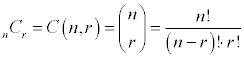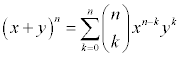# 7.5 - The Binomial Theorem

## Binomials raised to a power

A binomial is a polynomial with two terms. We're going to look at the Binomial Expansion Theorem, a shortcut method of raising a binomial to a power.

(x+y)0 = 1
(x+y)1 = x + y
(x+y)2 = x2 + 2xy + y2
(x+y)3 = x3 + 3x2y + 3xy2 + y3
(x+y)4 = x4 + 4x3y + 6x2y2 + 4xy3 + y4
(x+y)5 = x5 + 5x4y + 10x3y2 +10x2y3 + 5xy4 + y5

There are several things that you hopefully have noticed after looking at the expansion

• There are n+1 terms in the expansion of (x+y)n
• The degree of each term is n
• The powers on x begin with n and decrease to 0
• The powers on y begin with 0 and increase to n
• The coefficients are symmetric

## Pascal's Triangle

Pascal's Triangle, named after the French mathematician Blaise Pascal is an easy way to find the coefficients of the expansion.

Each row in the triangle begins and ends with 1. Each element in the triangle is the sum of the two elements immediately above it.

```              1
1   1
1   2   1
1   3   3   1
1   4   6   4   1
1   5  10  10   5   1
1   6  15  20  15   6   1
1   7  21  35  35  21   7   1
```

## Combinations

Combinations will be discussed more fully in section 7.6, but here is a brief summary to get you going with the Binomial Expansion Theorem.A combination is an arrangement of objects, without repetition, and order not being important. Another definition of combination is the number of such arrangements that are possible.

The n and r in the formula stand for the total number of objects to choose from and the number of objects in the arrangement, respectively.

The quickest way, if unimaginative, is to use the combination function on your calculator. On the TI-82 and TI-83, It is found under the Math menu, Probability submenu, choice 3. Enter the value for n first, then the nCr notation, then the value for r.

Each element in Pascal's Triangle is a combination of n things. The value for r begins with zero and works its way up to n. Or, because of symmetry, you could say it begins with n and works its way down to 0.

Let's consider the n=4 row of the triangle.

4C0 = 1, 4C1 = 4, 4C2 = 6, 4C3 = 4, 4C4 = 1

Notice that the 3rd term is the term with the r=2. That is, we begin counting with 0. This will come into play later.

## Binomial Expansion Theorem

Okay, now we're ready to put it all together.

The Binomial Expansion Theorem can be written in summation notation, where it is very compact and manageable.Remember that since the lower limit of the summation begins with 0, the 7th term of the sequence is actually the term when k=6.

When you go to use the binomial expansion theorem, it's actually easier to put the guidelines from the top of this page into practice. The x starts off to the nth power and goes down by one each time, the y starts off to the 0th power (not there) and increases by one each time. The coefficients are combinations.

### Binomial Expansion Example:

Expand ( 3x - 2y )5

Start off by figuring out the coefficients. Remember that these are combinations of 5 things, k at a time, where k is either the power on the x or the power on the y (combinations are symmetric, so it doesn't matter).

C(5,0) = 1; C(5,1) = 5; C(5,2) = 10; C(5,3) = 10; C(5,4) = 5; C(5,5) = 1

Now throw in the 3x and -2y terms.

1(3x)5(-2y)0 + 5(3x)4(-2y)1 + 10(3x)3(-2y)2 + 10(3x)2(-2y)3 + 5(3x)1(-2y)4 + 1(3x)0(-2y)5

Raise the individual factors to their proper powers.

1(243x5)(1) + 5(81x4)(-2y) + 10(27x3)(4y2) + 10(9x2)(-8y3) + 5(3x)(16y4) + 1(1)(-32y5)

Simplify each term to get the final answer.

243x5 - 810x4y + 1080x3y2 - 720x2y3 + 240xy4 - 32y5

### Finding the kth term

Find the 9th term in the expansion of (x-2y)13

Since we start counting with 0, the 9th term is actually going to be when k=8. That is, the power on the x will 13-8=5 and the power on the -2y will be 8. The coefficient is either C(13,8) or C(13,5), combinations are symmetric, so it doesn't matter.

C(13,8) * (x)5 (-2y)8 = 1287 (x5) (256y8) = 329472x5y8